Courses

# NABARD Manager Quant Aptitude Mock Test 6

## 20 Questions MCQ Test NABARD Manager - Mock Tests & Previous Year Papers | NABARD Manager Quant Aptitude Mock Test 6

Description
This mock test of NABARD Manager Quant Aptitude Mock Test 6 for Banking Exams helps you for every Banking Exams entrance exam. This contains 20 Multiple Choice Questions for Banking Exams NABARD Manager Quant Aptitude Mock Test 6 (mcq) to study with solutions a complete question bank. The solved questions answers in this NABARD Manager Quant Aptitude Mock Test 6 quiz give you a good mix of easy questions and tough questions. Banking Exams students definitely take this NABARD Manager Quant Aptitude Mock Test 6 exercise for a better result in the exam. You can find other NABARD Manager Quant Aptitude Mock Test 6 extra questions, long questions & short questions for Banking Exams on EduRev as well by searching above.
QUESTION: 1

Solution:
QUESTION: 2

### A park is in form of a rectangle 120 m x 100m. At the centre of the park is a circular lawn. The area of the park excluding the lawn is 10614 m2. The cost of grass carpeting the lawn @ Rs. 27/m2 is

Solution: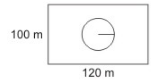Area of the park = 100 x 120
= 12000 m2
Area of the park excluding the lawn = 10614 m2
∴ The Area of the lawn = 12000 - 10614 = 1386 m2
The Cost of fencing the lawn = Rs. (27 x 1386) = Rs. 37422.00

QUESTION: 3

### Three candidates in an election received 1136,7636 and 11628 votes respectively. What percentage of the total votes did the winning candidate get?

Solution:
QUESTION: 4
In an expeiment,the average of 11 observations was 90. If the average of the first 5 observations is 87, and that of the last 5 is 84,then the sixth observation is
Solution:
QUESTION: 5
How many kg of salt costing Rs. 4 per kg must be mixed with 16 kg of salt costing Rs. 5.5 per kg so that 25 percent may be gained by selling the mixture Rs. 6 per kg?
Solution:
QUESTION: 6

A and B throw a dice. The probability that A's throw is not greater than B's is

Solution: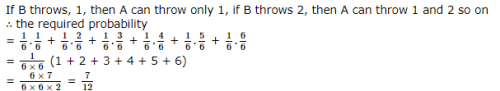QUESTION: 7
Which is the Number that comes next in the series:
2, 1, (1/2), (1/4), .?
Solution: This is a simple division series; each number is one-half of the previous number.
QUESTION: 8

A shopkeeper sold an article offering a discount of 5% and earned a profit of 23.5%. What would have been the percentage of profit earned if no discount was offered?

Solution:

Let C.P. be Rs 100
Then, S.P. = Rs 123.50
Let marked price be Rs x
Then, 95 100 x = 123.50
⇒ x = Rs ( 12350 /95 ) = Rs 130
Now, S.P. = Rs 130, C.P. = Rs 100
∴ Profit% = 30%

QUESTION: 9

Ratio of the incomes of A and B is 3:5 respectively and that of C and B is 9:7 respaectively. If the difference between the incomes of A and C is Rs 4,800, what is C's income?

Solution:
QUESTION: 10

Nikhal invested certain amount in three different schemes A, B and C with the rate of interest 10% per annum, 12% per annum and 15% per annum, respectively. If the total interest accrued in one yearwas Rs. 3200 and The amount invested in scheme C was 150% of the amount invested in scheme A and 240% of the amount invested in scheme B, what was the amount invested in scheme B ?

Solution:

Ratio of Nikhil's investment in difference schemes
= 100 : 150 x 100 /240 : 150 = 8 : 5 : 12
Now, according to the question,
8K x 10 /100 + 5Kx 12/ 100 + 12K x 15 /100 = 3200
or, 80K + 60K + 180K = 3200 x 100
or, 320K = 3200x 100
or K = 1000
∴ Amount invested in scheme B = 1000 x 5
= Rs 5000

QUESTION: 11

A tyre has two punctures. The first puncture alone would have made the tyre flat in 9 minutes and the second alone would have done it in 6 minutes. If air leaks out at a constant rate, how long does it take both the punctures together to make it flat?

Solution:

Required time = 9 x 6 /9 + 6 = 54/ 15
= 18/ 5 = 3.6 minutes

QUESTION: 12

The volumes of two spheres are in the ratio 8 : 27. The ratio of their surface areas is

Solution:
QUESTION: 13

In the following questions two equations numbered I and II are given. You have to solve both the equations and

I. √xx - √6√x6x = 0
II. y3 - 6(3232) = 0

Solution: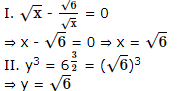QUESTION: 14

In the following questions two equations numbered I and II are given. You have to solve both the equations and

I. 3x - 2y = 10
II. 5x - 6y = 6

Solution:

By equation I � 3 - equation II,
9x - 6y - 5x + 6y = 30 - 6
⇒ 4x = 24 ⇒ x = 6
From equation I,
3 � 6 - 2y = 10
⇒ 2y = 18 - 10 = 8
⇒ y = 4

QUESTION: 15

Study the following graph carefuly and answer the questions given below it.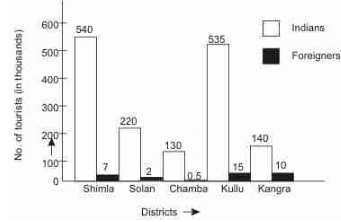How many districts in Himachal Pradesh were visitedby more than 10% of the total Indian tourists ?

Solution:

Total Indian tourists = 540 + 220 + 130 + 535 + 140 = 1565 thousand
10% of Indian tourists = (1565 � 10)100(1565 � 10)100 = 156.5 thousand

QUESTION: 16

Study the following graph carefuly and answer the questions given below it.By what percentage the Indian tourists visiting Chamba were less than those visiting Shimla ?

Solution:

Required % (540 - 130) 540 � 100 = 75%

QUESTION: 17

Study the diagram given below and answer these questions.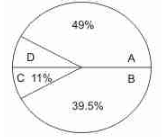A = Raw material cost
B = Packing material cost
C = Fixed manufacturing expenses
D = Labour cost

If the total value in rupees of all the sectors is Rs.128.3 lakh, then calculate the value of D in rupees,

Solution:

D represents = 0.5%
share of D = 128.3 � 0.5%
= 0.6 lakh (approximately)

QUESTION: 18

Study the diagram given below and answer these questions.A = Raw material cost
B = Packing material cost
C = Fixed manufacturing expenses
D = Labour cost

If the total cost of production doubles in a period of one year, then what will be the value of D?

Solution:

The value of D would be approximately = Rs 1.3 lakh

QUESTION: 19

In a Class X Board examination, ten papers are distributed over five Groups - PCB, Mathematics, Social Science, Vernacular and English. Each of the ten papers is evaluated out of 100. The final score of a student is calculated in the following manner. First, the Group Scores are obtained by averaging marks in the papers within the Group. The final score is the simple average of the Group Scores. The data for the top ten students are presented below. (Dipan's score in English Paper II has been intentionally removed in the table.)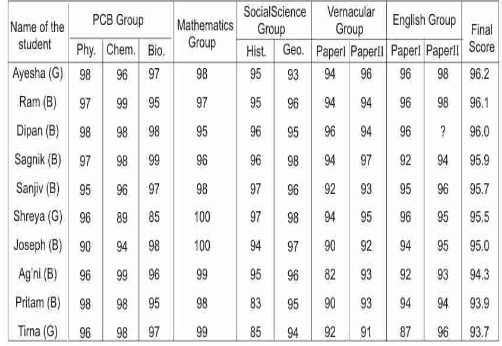Note : B or G against the name of a student respectively indicates whether the student is a boy or a girl.

How much did Dipan get in English Paper II ?

Solution:

Let marks obtained by Dipan in English group paper II be x, then group average of Dipan:
Physics, Chemistry and Biology group = 98
Maths = 95
Social Science = 95.5
Vernacular group = 95
English group = 96 + x/ 296 + x2
Now, final score = 98 + 95 + 95.5 + 95 +96 + x/2598 + 95 + 95.5 + 95 +96 + x/25
⇒ 96 = 767 + (96 + x)10767 + (96 + x)10 = 863 + x10863 + x10
or 960 = 863 + x
or 960 = 863 + x
or x = 97

QUESTION: 20

In a Class X Board examination, ten papers are distributed over five Groups - PCB, Mathematics, Social Science, Vernacular and English. Each of the ten papers is evaluated out of 100. The final score of a student is calculated in the following manner. First, the Group Scores are obtained by averaging marks in the papers within the Group. The final score is the simple average of the Group Scores. The data for the top ten students are presented below. (Dipan's score in English Paper II has been intentionally removed in the table.)Note : B or G against the name of a student respectively indicates whether the student is a boy or a girl.

Students who obtained Group Scores of at least 95 in every group are eligible to apply for a prize. Among those who are eligible, the student obtaining the highest Group Score in Social Science Group is awarded this prize. The Prize was awarded to :

Solution:

Group total of Shreya in Physics, Chemistry and Biology group is 90
Group total of Ram in Vernacular group is 94
Group total of Ayesha in Social Science is 94
Group total of Dipan in all the subjects is 95 or more